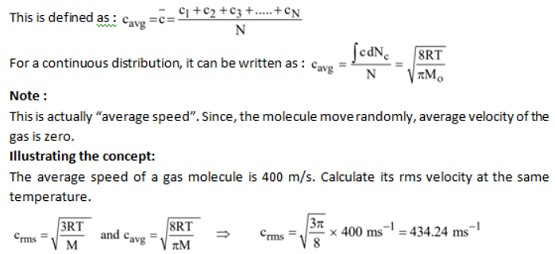# Formula For Average Speed of Gas Molecules

Sep 06, 2022, 16:45 IST

## Average speed (c­avg)

### What is the Average speed of gas molecules

As per the kinetic theory of gases, each molecule is moving with an altogether different velocity. Let ‘n’ molecules be present in a given mass of gas, in which n1 molecules have a speed of v1, n2 molecules have a speed of v2, ,nn molecules have a speed of vn. For More Chemistry Formulas just check out main page of Physics Wallah.

### i.e. Average velocity vavg = √8RT/πM

Where, R = gas constant

T= Temperature

M= molar mass

since R, M and pie are constant